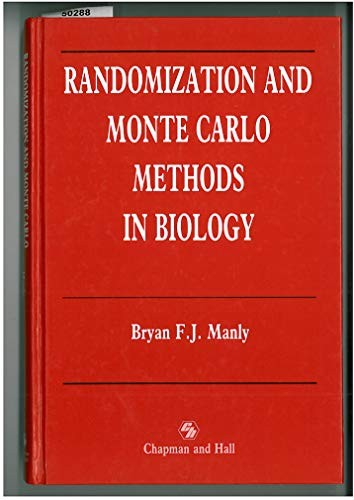# Randomization and Monte Carlo Method by Bryan Manly

Out of Stock
\$27.99
inc. GST
A discussion of the value of modern computer intensive methods for a wide range of statistical problems, with emphasis on biological applications. The book covers the applications to analysis of variance and regression multivariate methods.
Only 0 left

## Randomization and Monte Carlo Method Summary

### Randomization and Monte Carlo Method by Bryan Manly

This book aims to illustrate the value of modern computer intensive methods for a wide range of statistical problems. There is coverage of randomization methods of inference that were first proposed by R.A. Fisher in 1935, but are only now becoming practical because of the ready availability of computer power. Applications to analysis of variance and regression multivariate analysis are covered. Monte Carlo methods, jacknifing and bootstrapping are also discussed as being alternatives to randomization in some circumstances. The examples used have been chosen to attract interest and include the controversial topics of whether there is periodicity in fossil extinction records and whether there is evidence for a relationship between species co-occurences on islands. The first chapter introduces the ideas of randomization methods through some examples and the second introduces Monte Carlo tests and also the computer intensive techniques of jacknifing and bootstrapping since these are sometimes used as alternatives to randomization. The following chapters discuss some general theoretical matters concerning randomization and Monte Carlo methods and the analysis of various standard types of data. The final chapter gives some examples of non-standard situations where computer intensive methods seem to provide the best method for statistical analysis. In all chapters the emphasis is on applications in biology.

### Why buy from World of BooksOur excellent value books literally don't cost the earthFree delivery in AustraliaEvery used book bought is one saved from landfill

Part 1 Randomization tests and confidence intervals: the idea of a randomization test; examples of a randomization test; aspects of randomization testing raised by the examples; confidence intervals from randomization. Part 2 Monte Carlo and other computer intensive methods: Monte Carlo tests; jackknifing; bootstrapping; bootstrap tests of significance and confidence intervals. Part 3 Some general considerations: power; determining how many randomizations are needed; determining a randomization distribution exactly; the computer generation of pseudo-random numbers; generating random permutations. Part 4 One and two sample tests: the paired comparisons design; the one sample randomization test; the two sample randomization test; the comparison of two samples on multiple measurements. Part 5 Analysis of variance: one factor analysis of variance; Bartlett's test for constant variance; examples of more complicated types of analysis of variance; discussion; computer program. Part 6 Regrssion analysis: simple regression; testing for a non-zero beta value; confidence limits for beta; multiple linear regression; randomizing X variable values. Part 7 Distance matrices and spatial data: testing for association between distance matrices; Mantel's test; determining significance by sampling randomization distribution; confidence limits for a matrix regression coefficient; problems involving more than two matrices. Part 8 Other analyses on spatial data: the study of spatial point patterns; Mead's randomization test; a test based on nearest neighbour distances; testing for an association between two point patterns; the Besag-Diggle test; tests using distances between points. Part 9 Time series: randomization and time series; randomization tests for serial correlation; randomization tests for trend; randomization tests for periodicity; irregularly spaced series; tests on times of occurence; discussion of procedures for irregular series; bootstrap and Monte Carlo tests. Part 10 Multivariate data: univariate and multivariate tests; sample means and covariance matrices; comparison on sample means vectors; chi-squared analyses for count data; principal component analysis and other one sample methods; discriminate function analysis. Part 11 Ad hoc methods: the construction of tests in non-standard situations; testing for randomness of species co-occurences on islands; examining time change in niche ovelap; probing multivariate data with random skewers; other examples. Part 12 Conclusion: randomization methods; bootstrap and Monte Carlo methods.

GOR010429139
Randomization and Monte Carlo Method by Bryan Manly
Bryan Manly
Used - Like New
Hardback
Chapman and Hall
1990-10-31
292
0412367106
9780412367106
N/A
Book picture is for illustrative purposes only, actual binding, cover or edition may vary.
The book has been read, but looks new. The book cover has no visible wear, and the dust jacket is included if applicable. No missing or damaged pages, no tears, possible very minimal creasing, no underlining or highlighting of text, and no writing in the margins.